# 2.灵感代办

• 浪费100块钱，周六日加班上课补回来

# 3.学习内容

• ## 异或 XOR

• 相同为0，不同为1
• 与自身异或 为0
• 与零异或 为本身
• 交换律 a\^b\^c = c\^b^a
• ## 理论分析

• 算法就是对操作的统计
• 一次赋值操作，判断操作，都是操作
• 比如一个for 赋值3次，循环n次 就是3n
• 一个循环三次里面的赋值操作 就是3n-1
• ## 算法三大特性（五大特性）

• 输入
• 0或多个输入
• 输出，
• 一个多个输出
• 有穷性
• 有限的步骤，有限的时间
• 确定性
• 每一步都有确定的含义，没有二义性
• 可行性
• 能够用计算机实现出来
• ## 算法的定义

• 算法是独立存在的一种解决问题的方案和思想
• 算法的实现语言不重要，重要的是思想
• ## Python的Timeit模块

• timeit.Timer(stmt=‘pass’,setup=’pass’,timer=’function)
• Timer是测试小段代码执行速度的类
• stmt参数是要测试的语句 statment
• setup参数是运行代码需要的设置，比如循环，导入包
• timer参数是定时器函数，与平台有关
• timeit.timer.timeit(number=10000)
• 对代码执行多少次的平均值
• ## 大O 计数法

• T(n) 时间复杂度
• T(n)=n^3 *2 和 T(n)=3^3 *10 共性就是T = n^3

# 4.扩展延伸知识

• ## 初中数学

• ### 实数

•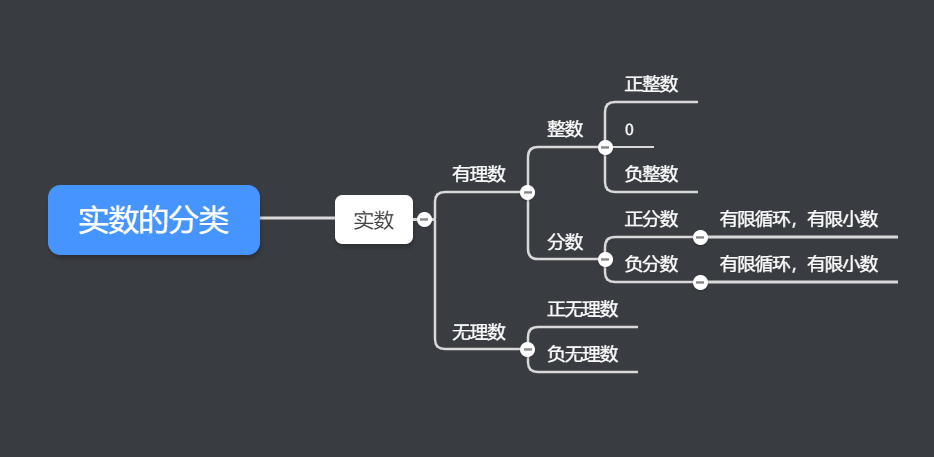• 实数的运算顺序
• 先乘方，开方，在乘除，最后加减，有括号先算括号
• ${a}^{0}=1\left(a!=1\right)（a\ne 0\right)$

,

${a}^{\left(-p\right)}=\frac{1}{{a}^{p}}\phantom{\rule{1em}{0ex}}a\ne 0\phantom{\rule{1em}{0ex}},p是正数$

• ### 相反数

• a的相反数是-a，相反数相加为0
• ### 倒数

• a和b互为倒数，那么a*b=1

• 正数绝对值为正数
• 负数绝对值为正数
• ### 科学计数法

• 科学计数法的数字在1-10之间，1<=a<10
• 正数是

${9}^{n}$

,负数是

${9}^{-n}$

• ### 幂的运算

•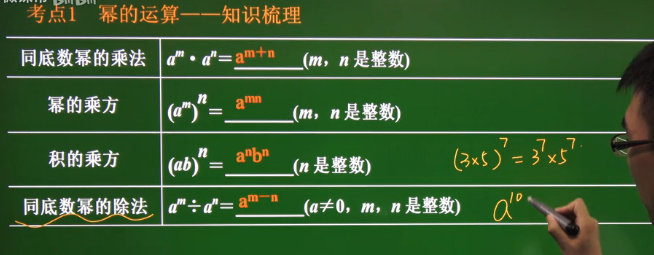• ### 乘法公式

•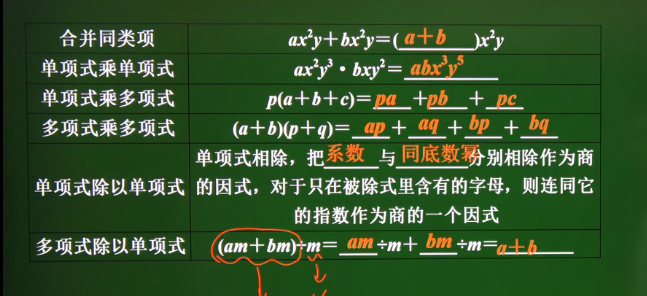• ### 整式的运算

•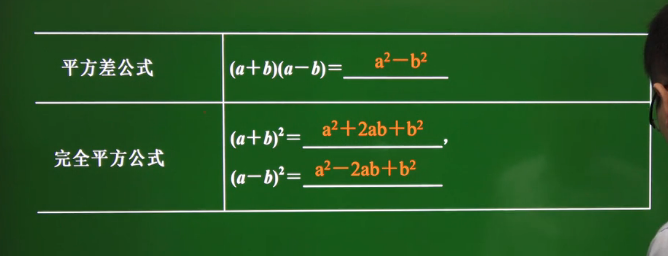• ### 因式分解

•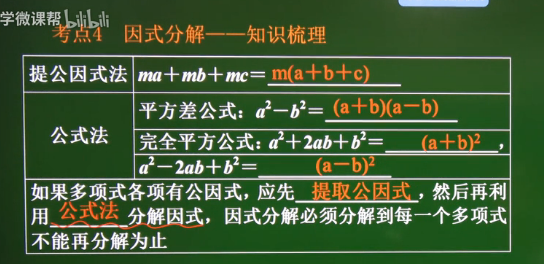•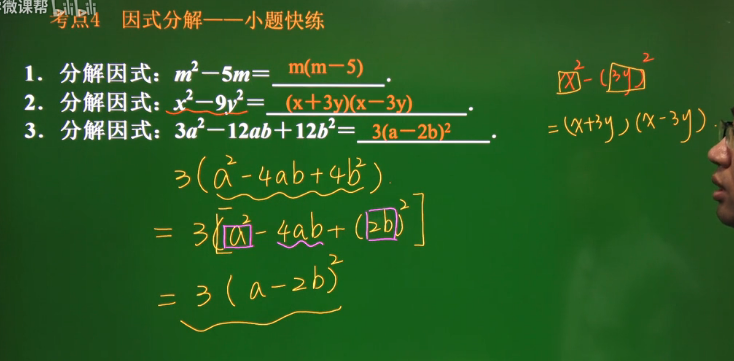Ares个人进阶之路